### SOLUTIONS TO INTEGRATION BY PARTS

SOLUTION 10 : Integrate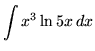. Letandso thatand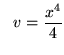.

Therefore,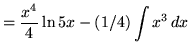.

SOLUTION 11 : Integrate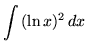. Letandso thatand.

Therefore,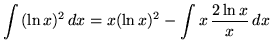.

Use integration by parts again. Let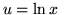andso that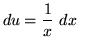and.

Hence,.

SOLUTION 12 : Integrate. Let

einandso thatand.

Therefore,.

Use integration by parts again. Letandso thatand.

Therefore,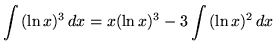.

Use integration by parts for a third time. Letandso thatand.

Hence,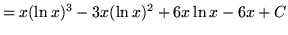.

SOLUTION 13 : Integrate. Let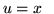andso thatand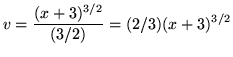.

Therefore,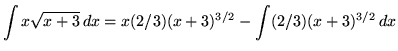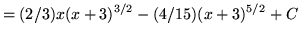.

SOLUTION 14 : Integrate. Letandso thatand.

Therefore,.

Now use the trig identity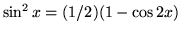,

getting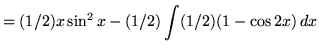.

SOLUTION 15 : Integrate. Letandso thatand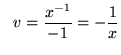.

Therefore,.

Use integration by parts again. Letand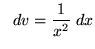so thatand.

Hence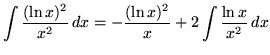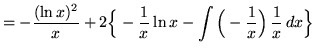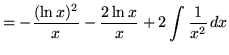.